Deviant Art Shop Forum More Submit Join Login

# LOVE IS LOVE

Made with pride by the DeviantArt community

##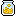Donate

has started a donation pool!
614 / 1,200
 oh

You must be logged in to donate.

## Comments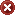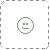Add a Comment: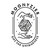Featured By Owner May 8, 2019  Professional Artisan Crafter
Thank you for the support, I'm glad you liked our crafts!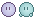!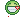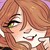Featured By Owner Apr 8, 2019  Hobbyist Digital Artist
<3 <3 <3 <3 <3 <3 <3 <3 <3<3 <3 <3 <3 <3 <3 <3 <3 <3<3 <3 <3 <3 <3 <3 <3 <3 <3<3 <3 <3 <3 <3 <3 <3 <3 <3<3 <3 <3 <3 <3 <3 <3 <3 <3<3 <3 <3 <3 <3 <3 <3 <3 <3<3 <3 <3 <3 <3 <3 <3 <3 <3<3 <3 <3 <3 <3 <3 <3 <3 <3<3 <3 <3 <3 <3 <3 <3 <3 <3<3 <3 <3 <3 <3 <3 <3 <3 <3<3 <3 <3 <3 <3 <3 <3 <3 <3<3 <3 <3 <3 <3 <3 <3 <3 <3<3 <3 <3 <3 <3 <3 <3 <3 <3<3 <3 <3 <3 <3 <3 <3 <3 <3<3 <3 <3 <3 <3 <3 <3 <3 <3<3 <3 <3 <3 <3 <3 <3 <3 <3<3 <3Featured By Owner Apr 8, 2019  Hobbyist Digital Artist
love u <3 <3 <3 <3 <3 <3 <3 <3 <3<3 <3 <3 <3 <3 <3 <3 <3 <3<3 <3 <3 <3 <3 <3 <3 <3 <3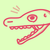Featured By Owner Apr 1, 2019  Hobbyist General Artist
>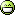DD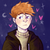Featured By Owner Mar 17, 2019  Student Digital Artist
Ogólnie
BOŻE DZIĘKUJE ZA POINTSY
KOCHAM
NAPRAWDĘ DZIĘKUJE UWUFeatured By Owner Mar 21, 2019  Hobbyist General Artist
<3 Polaczki muszą siebie wspierać uwuAdd a Comment: